# Generate Generic C/C++ for Sequence-to-Sequence Deep Learning Simulink Models

This example shows how to generate a generic C/C++ executable that does not depend on any third-party deep learning libraries from a deep learning Simulink® model. The example predicts the remaining useful life (RUL) of a turbofan engine, measured in cycles at each step of an input time series that represents data from various sensors in an engine.

For a video demonstration of this example, see Generate Generic C/C++ Code for Deep Learning Networks in Simulink.

This example is based on the Generate Generic C/C++ Code for Sequence-to-Sequence Regression That Uses Deep Learning example from MATLAB® Coder™. For more information, see Generate Generic C/C++ Code for Sequence-to-Sequence Regression That Uses Deep Learning.

#### Prerequisites

For PIL verification, you will need:

• STMicroelectronics STM32F407G-Discovery, STM32F746G-Discovery, or STM32F769I-Discovery board

• USB type A to Mini-B cable

• USB TTL-232 cable - TTL-232R 3.3V (serial communication for STM32F4-Discovery board)

• Embedded Coder® Support Package for STMicroelectronics Discovery Boards. To install this support package, use the Add-Ons menu in the Environment section of the MATLAB Home tab.

### Pretrained RUL Network

This example uses a pretrained LSTM network to predict the remaining useful life of an engine, measured in cycles. The LSTM network consists of an LSTM layer with 200 hidden units, followed by a fully connected layer of size 50 and a dropout layer with dropout probability 0.5.The network was trained using the Turbofan Engine Degradation Simulation Data Set as described in . The training data contains simulated time series data for 100 engines. Each sequence varies in length and corresponds to a full run to failure (RTF) instance. The test data contains 100 partial sequences and corresponding values of the remaining useful life at the end of each sequence. For more information on training the network, see the example Sequence-to-Sequence Regression Using Deep Learning (Deep Learning Toolbox).

`net = coder.loadDeepLearningNetwork('rulNetwork.mat')`
```net = SeriesNetwork with properties: Layers: [6×1 nnet.cnn.layer.Layer] InputNames: {'sequenceinput'} OutputNames: {'regressionoutput'} ```

For information on the networks, layers, and classes supported for code generation, see Networks and Layers Supported for Code Generation.

### Prepare Test Data

Load the `TurboFanRULValidate` MAT-file that contains the Turbofan Engine Degradation Validation Data Set. This MAT-file stores the variable `XValidate` that contains 100 sample observations representing sensor readings that you use to test the Simulink model.

`load('TurboFanRULValidate.mat')`

To make the input validation data compatible with Simulink code generation, the sequence lengths for each of the independent 100 observations are zero-padded to create uniformly-sized, 17-by-303 input arrays.

```sequenceLengths = cellfun(@length,XValidate,'UniformOutput',true); maxSequenceLen = max(sequenceLengths); padFcn = @(x) [x,zeros(size(x,1),maxSequenceLen-size(x,2))]; XValidatePad = cellfun(padFcn,XValidate,'UniformOutput',false);```

The padded values are then converted to a 17-by-303-100 numeric array. To import this data into the Simulink model, specify a structure variable containing the data values and an empty time vector. During simulation, the input for the first time step is read from the first 17-by-303 element of the array. The value for the second time step is read from the second element, and so on, for a total of 100 steps.

```simin.time = []; simin.signals.values = cell2mat(reshape(XValidatePad,1,1,[])); simin.signals.dimensions = size(XValidatePad{1});```

### Simulink Model for Prediction

The Simulink model for predicts the remaining useful life of a turbofan engine is shown. The model uses the `Predict` block from the `Deep Neural Networks` library that imports the trained network from a `rulNetwork` MAT-file. Additionally, the `Mini-batch size` parameter of the block is set to 1.

```model = 'rulPredict'; open_system(model)```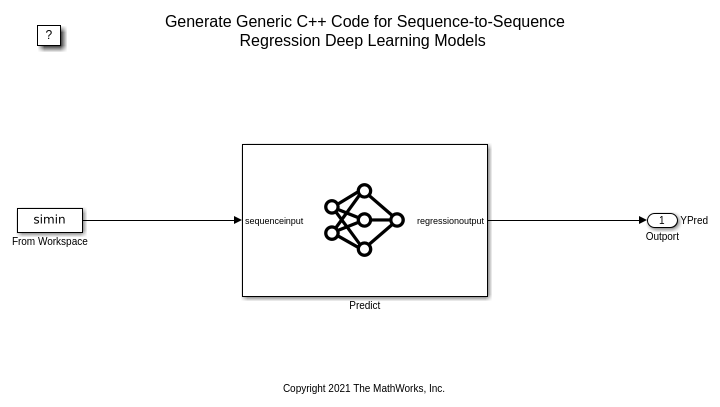### Run the Simulation

To validate the Simulink model, run the simulation.

```set_param(model,'SimulationMode','Normal'); sim(model);```

The output `YPred` of the Simulink model contains the predicted remaining useful life values from the network. This output is first trimmed to remove the results from the zero-padded values and then converted to a cell array.

```YPred_cell = squeeze(mat2cell(YPred,1,maxSequenceLen,ones(1,100))); for t = 1:length(sequenceLengths) YPred_cell{t}(:,sequenceLengths(t) + 1:end) = []; end ```

Plot the predicted remaining useful life (RUL) values for four randomly-selected observations and compare them to the validation data.

```observationIdx = randperm(100,4); rulExamplePlots(observationIdx,YValidate,YPred_cell);```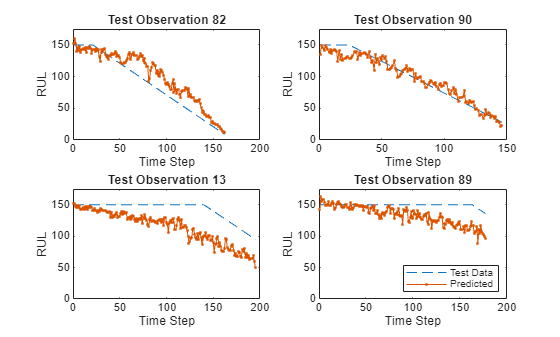### Configure Model for Code Generation

To generate C/C++ code that does not depend on any third-party deep learning libraries and build an executable, select the generic real-time (ERT) target from Embedded Coder™.

```cs = getActiveConfigSet(model); switchTarget(cs,'ert.tlc',[]);```

Configure code generation specific parameters. Setting MAT-file logging enables the generated code to redirect simulation data to a MAT-file.

```set_param(cs,'TargetLang','C'); set_param(cs,'Toolchain','Automatically locate an installed toolchain'); set_param(cs,'ObjectivePriorities','Execution efficiency'); set_param(cs,'DLTargetLibrary','None'); set_param(cs,'GenerateReport','on'); set_param(cs,'CodeInterfacePackaging','Nonreusable function'); set_param(cs,'MatFileLogging', 'on');```

### Generate and Build the Simulink Model

Generate and build the Simulink model by using the `slbuild` command. The code generator places the files in a build folder, a subfolder named rulPredict`_ert_rtw` under your current working folder.

`evalc("slbuild('rulPredict')");`

### Run the Generated Executable

Run the generated executable. After successful execution, the `rulPredict` executable creates a `rulPredict` MAT-file in the current working folder.

```if ispc status = system('rulPredict.exe'); else status = system('./rulPredict'); end ```
```** created rulPredict.mat ** ```
`load('rulPredict.mat')`

The output `rt_YPred` contains the predicted remaining useful life values from the network. This output is first trimmed to remove the results from the zero-padded values and then converted to a cell array.

```rt_YPred_cell = squeeze(mat2cell(rt_YPred,1,maxSequenceLen,ones(1,100))); for t = 1:length(sequenceLengths) rt_YPred_cell{t}(:,sequenceLengths(t) + 1:end) = []; end ```

Plot the predicted remaining useful life (RUL) values for four randomly-selected observations and compare them to the validation data.

`rulExamplePlots(observationIdx,YValidate,rt_YPred_cell);`### Code Verification with Processor-in-the-Loop (PIL) Simulation

To ensure that the behavior of the deployment code matches the design, you can configure the Simulink model to run a PIL simulation on embedded boards. In a PIL simulation, the generated code runs on embedded boards such as the STMicroelectronics® Discovery. The results of the PIL simulation are transferred to Simulink to verify numerical equivalence of the simulation and the code generation results.

This example shows PIL verification on the STMicroelectronics Discovery board by using the Embedded Coder® Support Package for STMicroelectronics Discovery Boards. This example is pre-configured to run on the STM32F746G-Discovery board. You can configure this model to use other supported embedded boards by selecting them as the "Hardware board" on the Hardware Implementation pane of the Model Configuration Parameters.

#### Prepare Test Data

For PIL simulation, this example sorts the observations by sequence length and picks the first 10. These observations are zero-padded to create uniformly-sized, 17-by-54 input arrays. The padded values are then converted to a 17-by-54-10 numeric array. To import this data into the Simulink model, specify a structure variable containing the data values and an empty time vector. During simulation, the input for the first time step is read from the first 17-by-54 element of the array. The value for the second time step is read from the second element, and so on, for a total of 10 steps.

```[~,idx] = sort(cellfun(@length,XValidate)); XValidatePIL = XValidate(idx(1:10)); YValidatePIL = YValidate(idx(1:10)); sequenceLengths = cellfun(@length,XValidatePIL,'UniformOutput',true); maxSequenceLen = max(sequenceLengths); padFcn = @(x) [x,zeros(size(x,1),maxSequenceLen-size(x,2))]; XValidatePILPad = cellfun(padFcn,XValidatePIL,'UniformOutput',false); simin.time = []; simin.signals.values = cell2mat(reshape(XValidatePILPad,1,1,[])); simin.signals.dimensions = size(XValidatePILPad{1});```

#### Top Model PIL

The `rulPredict_pil` is a modified version of the `rulPredict` model for PIL verification. To load the test vectors, the `rulPredict_pil` model replaces the `From Workspace` block with an `Inport` block. The `Inport` block is configured to accept a 17-by-54 array of double data type.

```pilModel = 'rulPredict_pil'; open_system(pilModel);```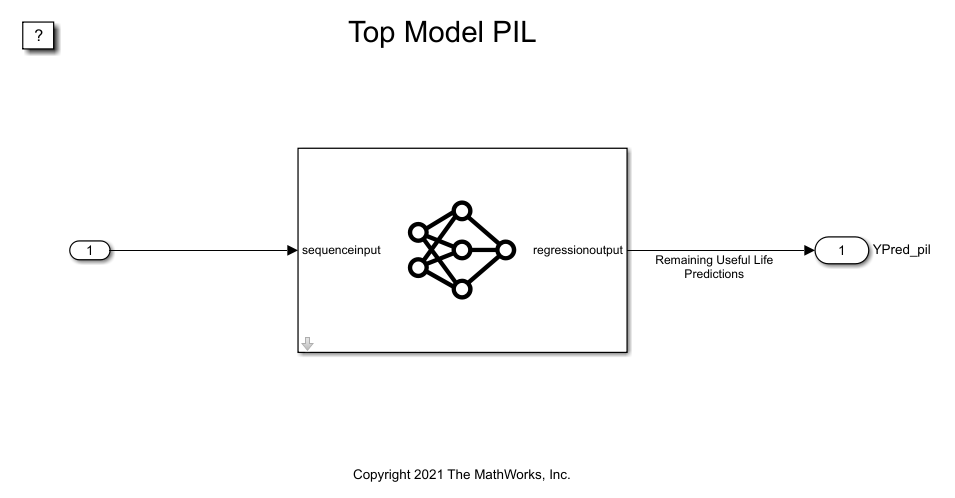Configure the `rulPredict_pil` model for the STM32F467G-Discovery target.

```cs = getActiveConfigSet(pilModel); set_param(cs,'HardwareBoard','STM32F746G-Discovery'); set_param(cs,'Toolchain','GNU Tools for ARM Embedded Processors'); ```

The STM32F4-Discovery board supports two different communication interfaces for PIL: ST-LINK and serial. The ST-LINK communication interface does not require any additional cables or hardware besides a USB type A to Mini-B cable used to connect the STM32F4-Discovery board to the host computer. The serial communication interface requires a USB TTL-232 cable. Running a PIL simulation using the serial communication interface is much faster than the running a PIL simulation using ST-LINK. This example is configured to use the serial interface.

Additionally, you must set the COM port parameter in Configuration Parameters > Hardware Implementation > Target Hardware Resources > PIL to match the port number of the serial interface on your Windows computer.

#### Run PIL Simulation

To compare the PIL results with simulation, run the `rulPredict_pil` model in normal mode and then in PIL mode.

```set_param(cs,'LoadExternalInput','on'); set_param(cs, 'ExternalInput','simin'); set_param(pilModel,'SimulationMode','Normal'); sim(pilModel); YPred_ref = YPred_pil; set_param(pilModel,'SimulationMode','Processor-in-the-Loop (PIL)') sim(pilModel); ```

#### Plot Results

The output `YPred_pil` and `YPred_ref` contain the predicted remaining useful life values from the network. This output is first trimmed to remove the results from the zero-padded values and then converted to a cell array.

Plot the predicted remaining useful life (RUL) values for PIL and compare them to the validation data.

```YPred_ref_cell = squeeze(mat2cell(YPred_ref,1,maxSequenceLen,ones(1,10))); YPred_pil_cell = squeeze(mat2cell(YPred_pil,1,maxSequenceLen,ones(1,10))); for t = 1:length(sequenceLengths) YPred_ref_cell{t}(:,sequenceLengths(t) + 1:end) = []; YPred_pil_cell{t}(:,sequenceLengths(t) + 1:end) = []; end rulExamplePlots([1:10],YValidatePIL,YPred_pil_cell); ```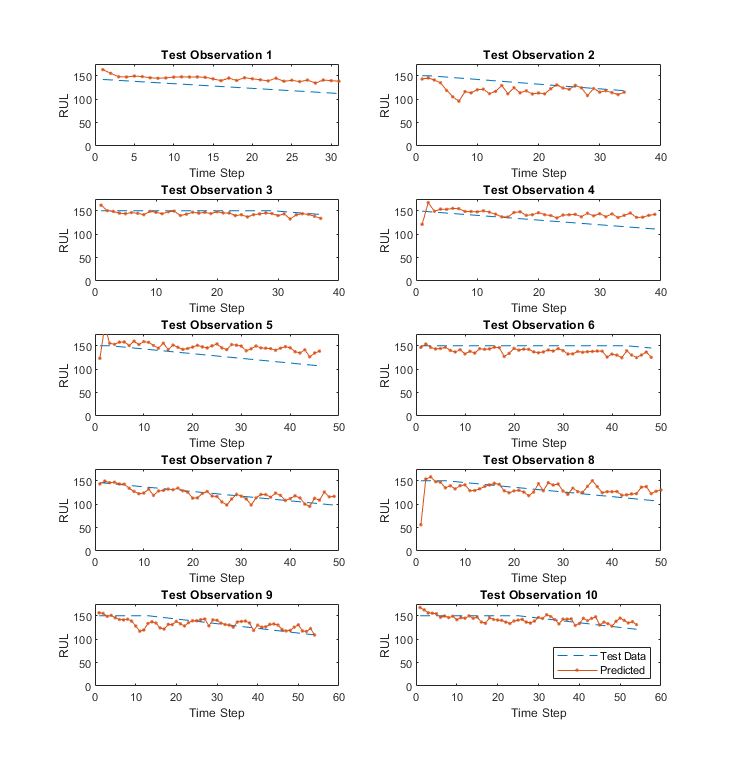Compute the error from the PIL simulation and plot the results.

```YPred_error = YPred_ref-YPred_pil; figure('Name', 'PIL Error') for i = 1:10 subplot(5,2,i) plot(YPred_error(:,:,i),'.-') maxerror = string(max(YPred_error(:,:,i))); ylim([-1e-4 1e-4]) title("Test Observation " + i) xlabel("Time Step") ylabel("Difference") end legend(["Difference in Simulation Modes"],'Location','southeast') ```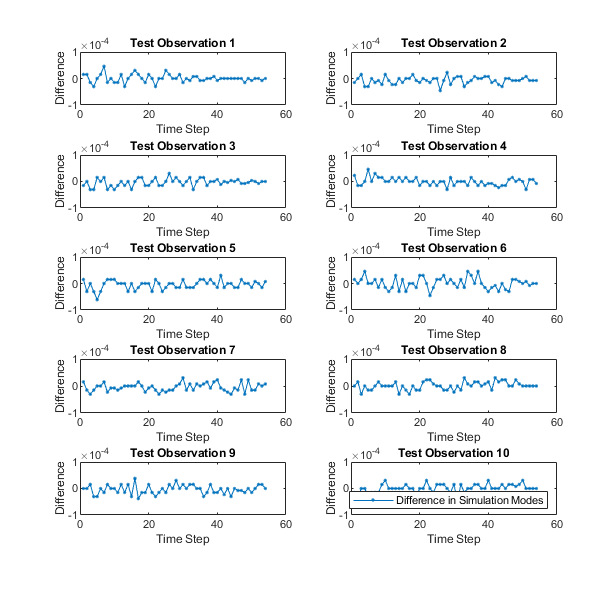### Supporting Functions

```function rulExamplePlots(observationIdx,YTest,YPred) N = numel(observationIdx); figure for i = 1:N subplot(N/2,2,i) plot(YTest{observationIdx(i)},'--') hold on plot(YPred{observationIdx(i)},'.-') hold off ylim([0 175]) title("Test Observation " + observationIdx(i)) xlabel("Time Step") ylabel("RUL") end legend(["Test Data" "Predicted"],'Location','southeast') end```

### References

1. Saxena, Abhinav, Kai Goebel, Don Simon, and Neil Eklund. "Damage propagation modeling for aircraft engine run-to-failure simulation." In Prognostics and Health Management, 2008. PHM 2008. International Conference on, pp. 1-9. IEEE, 2008.